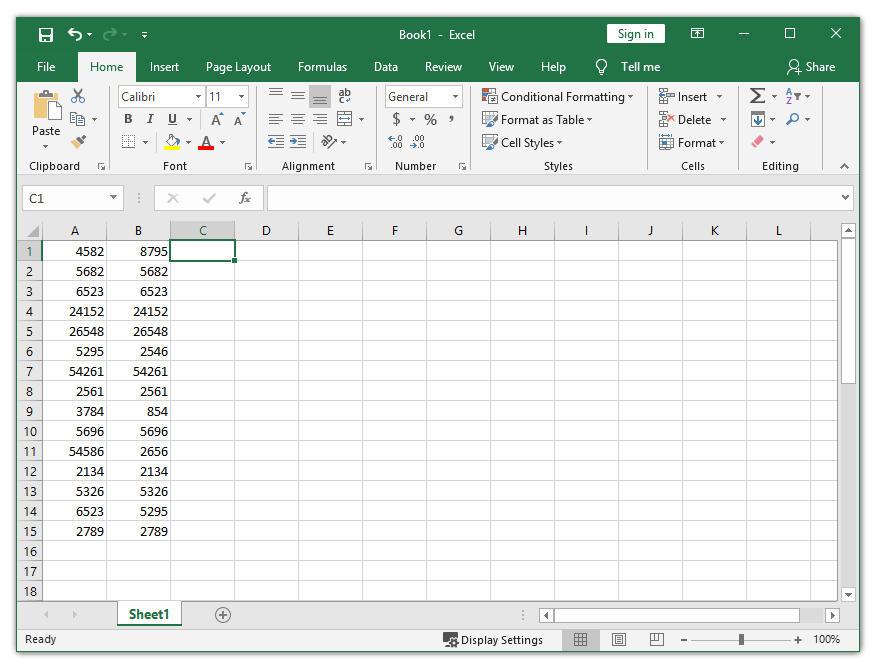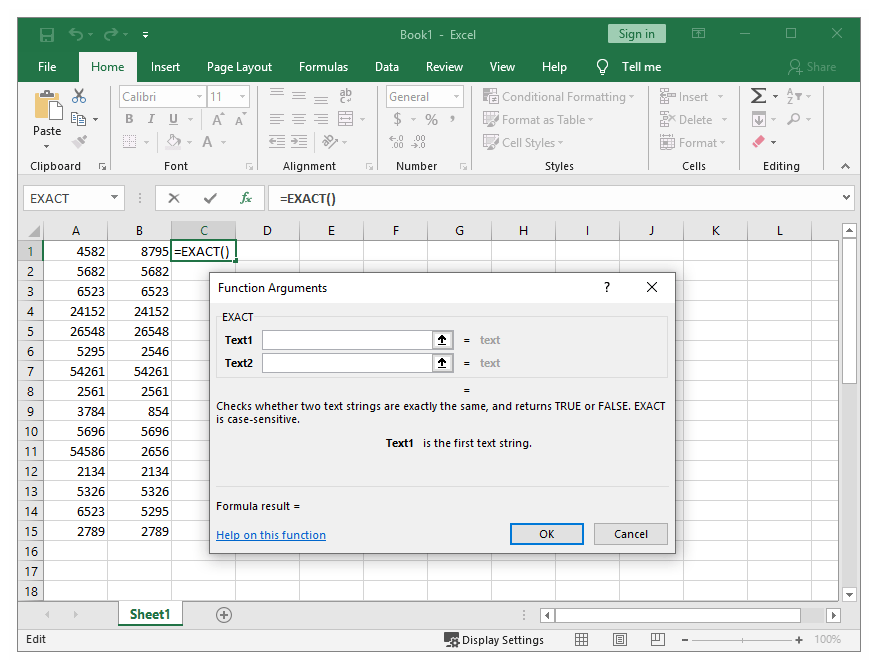0

# How To Tell if Two Cells in Excel Contain the Same ValueMany companies still use Excel as it allows them to store different types of data, such as tax records and business contacts.

Since everything is being done manually in Excel, there is a potential risk of storing false information. Maybe you make a typo or read a certain number wrong. Making these kinds of mistakes can sometimes lead to very serious consequences.

That’s exactly why accuracy is so important when working in Excel. Thankfully, people behind Excel have thought about this problem, so they have included features and tools that help everyday users check their data and correct the errors.

This article will show you how to check if two cells in Excel have the same value.

## Use the Exact Function

If you want to check whether or not two cells have the same value but don’t want to go through the whole table manually, you can make Excel do this for you. Excel has a built-in function called Exact. This function works for both numbers and text.

Here is how you can use the Exact Excel function.Let’s say that you are working with the worksheet from the picture. As you can see, it isn’t that easy to determine whether the numbers from column A are the same as the numbers from column B.

To make sure that cells from column A don’t have a duplicate in the corresponding column B cells, use the Exact function. For example, if you want to check cells A1 and B1, you can use the Exact function in the C1 cell.

When you use this function, Excel will return a value of TRUE if the numbers match, and the value of FALSE if they don’t.

To locate the Exact function, click on the Formulas tab and then select the Text button. Choose EXACT from the drop-drown menu. Although the Exact formula is defined as a text function (hold the cursor on Exact and you’ll see its definition), it works on numbers as well.

After clicking on EXACT, a window called Function Arguments will appear. In this window, you need to specify exactly which cells you want to compare.So, if you want to compare cells A1 and B1, type A1 in the Text1 box and then B1 in the Text2 box. After that, simply click OK.

As the numbers from cells A1 and B1 don’t match (check the previous picture), you will notice that Excel returned a FALSE value and stored the result in cell C1.

You don’t have to repeat the same steps in order to check all the other cells as that would be too much of a hassle. Instead, you can drag the fill handle, which is a small square located in the bottom-right corner of the cell, all the way down. This will copy and apply the formula to all the other cells.

After you have done that, you should notice the FALSE value in C6, C9, C11, and C14. The rest of the cells in the C column are marked as TRUE because the formula found a match.## Use the IF Function

Another function that allows you to compare two cells is the IF function. It compares the cells from a column row by row. Let’s use the same two columns (A1 and B1) as in the previous example.

To use the IF function correctly, you will need to remember its syntax.

In cell C1, type the following formula: =IF(A1=B1, “Match”, “ “)After executing this formula, Excel will put “Match” in the cell if the two values are identical.

On the other hand, if you want to check for differences, you should type the following formula: =IF(A1<>B1, “No match”,” “)

Excel also allows you to check for both matches and differences in the same formula. All that you need to do is type either =IF(A1<>B1, “No match”, “Match”) or =IF (A1=B1, “Match”, “No match“).

## Check for Duplicates and Correct Mistakes

These are the easiest methods to find out whether two cells have the same value in Excel. Of course, there are other, more complex methods that can do the same, but these two should be enough for everyday users.

Now that you know how to look for duplicates in your Excel worksheet, you can easily fix errors and make sure that you have entered the correct data.

Disclaimer: Some pages on this site may include an affiliate link. This does not effect our editorial in any way.